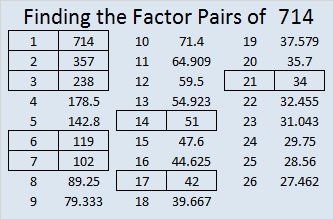# 714 Home Runs

• 714 is a composite number.
• Prime factorization: 714 = 2 x 3 x 7 x 17
• The exponents in the prime factorization are 1, 1, 1, and 1. Adding one to each and multiplying we get (1 + 1)(1 + 1)(1 + 1)(1 + 1) = 2 x 2 x 2 x 2 = 16. Therefore 714 has exactly 16 factors.
• Factors of 714: 1, 2, 3, 6, 7, 14, 17, 21, 34, 42, 51, 102, 119, 238, 357, 714
• Factor pairs: 714 = 1 x 714, 2 x 357, 3 x 238, 6 x 119, 7 x 102, 14 x 51, 17 x 42, or 21 x 34
• 714 has no square factors that allow its square root to be simplified. √714 ≈ 26.720778.Babe Ruth hit 714 home runs. His record for the most home runs stood for nearly 39 years when Hank Aaron hit the 715th home run of his career.

The number of prime factors that 714 has is a power of two so 714 can make a very attractive factor tree all by itself. 715 only has three prime factors so its factor tree doesn’t look as nice. I still wanted to make factor trees for both of them so I multiplied them together and made this impressive looking factor tree:(The picture of the 714 baseball in the graphic above listed e-bay as the source while the picture of the 715 baseball was from Sports Memorabilia.com. The 715 ball is autographed by Al Downing, the former all-star and comeback player of the year, who pitched the ball that became Hank Aaron’s 715th home run.)

The numbers 714 and 715 form what is now called a Ruth Aaron Pair. Other consecutive numbers whose factors add up to equal numbers are also given that name.

Between the numbers 714 and 715 ALL the prime numbers less than or equal to 17 are used as factors exactly one time.

I became aware of these facts several months ago when Dan Bach sent me this tweet:

This is my 714th post so I will focus on the number 714 and some different ways it hits a home run:

714 can be written as the sum of consecutive numbers many ways:

• 237 + 238 +239 = 714; that’s 3 consecutive numbers.
• 177 + 178 + 179 + 180 = 714; that’s 4 consecutive numbers.
• 99 + 100 + 101 + 102 + 103 + 104 + 105 = 714; that’s 7 consecutive numbers.
• 54 + 55 + 56 + 57 + 58 + 59 + 60 + 61 + 62 + 63 + 64 + 65 = 714; that’s 12 consecutive numbers.
• 34 + 35 + 36 + 37 + 38 + 39 + 40 + 41 + 42 + 43 + 44 + 45 + 46 + 47 + 48 + 49 + 50 = 714; that’s 17 consecutive numbers.
• 24 + 25 + 26 + 27 + 28 + 29 + 30 + 31 + 32 + 33 + 34 + 35 + 36 + 37 + 38 + 39 + 40 + 41 + 42 + 43 + 44 = 714; that’s 21 consecutive numbers.

714 is the sum of the twelve prime numbers from 37 to 83.

714 is also the sum of the fourteen prime numbers from 23 to 79. Can you name all those prime numbers?

Because 17 is one of its factors, 714 is the hypotenuse of the Pythagorean triple 336-630-714. What is the greatest common factor of those three numbers?

Stetson.edu alerted me to the fact that 714 is the smallest number that uses all possible digits exactly the same number of times in both BASE 2 and BASE 5:

• 714 is 1011001010 in BASE 2; that’s five 1’s and five 0’s, the only digits used in BASE 2.
• 714 is 10324 in BASE 5; note that 0, 1, 2, 3, and 4 are the only digits used in BASE 5, and each is used exactly once.

In addition 714 is a palindrome in two bases:

• 181 BASE 23; note that 1(23²) + 8(23) + 1(1) = 714.
• LL BASE 33 (L is 21 in base 10); note that 21(33) + 21(1) = 714.

After I wrote this post, I tweeted Dan Bach about it, and he let me know how he first learned about Ruth Aaron pairs: